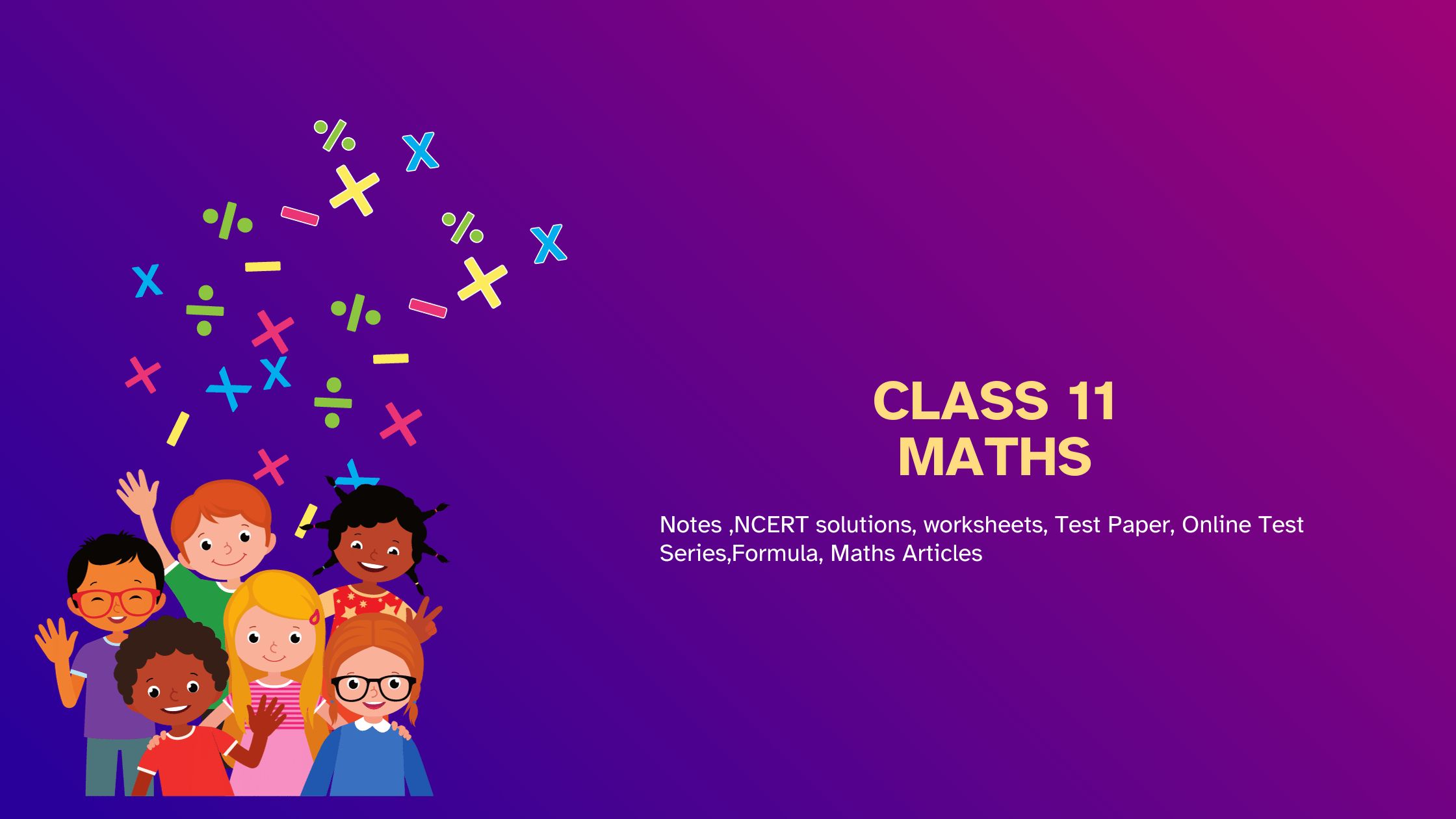# Class 11 Maths NCERT Solutions and Notes

•• Master Class 11 Maths And Be Successful in exams.Here find Class 11 Maths Notes, assignments,NCERT Solutions concept maps and lots of study material for easy learning and understanding. We have lots of study material written in easy language that is easy to follow.

1. First read and understand the notes.
2. Try to go through the solved examples of of the chapter you are reading
3. Now it is the time to solve unsolved assignments and worksheets. You will find plenty of them here or on the respective chapter page.
All the best for better learning of your maths concepts. Hope we helped you when you were looking for class 11 Maths notes .

Notes
Assignment
NCERT Solutions

Notes
Assignments
NCERT Solutions

Notes
Assignments

## Chapter 4: Complex numbers And Quadratic equation

Notes
NCERT Solutions Assignments

Notes
NCERT Solutions

Notes
NCERT Solutions

Notes

Notes

Notes

Notes

Notes

Notes
NCERT Solutions

Notes

Notes
Assignments

## Frequently asked Questions on CBSE Class 11 Maths

### How many chapters are there in Class 11 Maths?

There are total 14 chapters in Class 12 Maths as per the rationalized Syllabus. All the chapters are required for assessment

### What is the best books for Class 11 Maths?

Couple of book
(a)NCERT Class 11 Maths textbook
(b)NCERT Class 11 Exemplar
(c)R D Sharma

### Which is the easiest chapter of class 11 Maths NCERT Text book?

Introduction to Three-dimensional Geometry and Sets are the easiest chapter

### Is Class 11 Maths NCERT Text book Sufficient for CBSE Exams?

It is sufficient if you go through all the questions in the NCERT text book thoroughly and practice them all.However to enhance your maths skill , you can go for NCERT exemplar and other books

### what is the best way to learn the concept in Class 11 Maths?

First go through all the Notes and solved examples and Then do the exercises. Practice Lot of questions. The simple Mantra is Practice .Practice and Practice

### what is the deleted syllabus in Class 11 Maths?

Chapter Sets
Power sets
Chapter Trigonometric Functions
General solution of trigonometric equations of the type sin y = sin a, cos y = cos a and tan y = tan a.
Chapter : Mathematical Induction
Full chapter
Chapter : Complex Numbers
Polar representation of complex numbers. Statement of Fundamental Theorem of Algebra, solution of quadratic equations (with real coefficients) in the complex number system. Square root of a complex number.
Chapter : Linear inequalities
Graphical solution of linear inequalities in two variables. Graphical method of finding a solution of system of linear inequalities in two variables.
Chapter : Binomial Theorem
General and middle term in binomial expansion
Chapter : Sequence and Series
Arithmetic Progression, Sum to n terms of Special Series
Chapter : Straight line
Intercept normal form. General equation of a line. Equation of family of lines passing through the point of intersection of two lines.
Chapter : Introduction to Three-dimensional Geometry
Section Formula
Chapter : Mathematical Reasoning
Full chapter
Chapter : Statistics
Analysis of frequency distributions with equal means but different variances.
Chapter : Probability
Random experiments; outcomes, sample spaces (set representation).